# Normalizing fastai Tabular Inputs

technical
Exploring the effectiveness of normalization on tabular data
Author

Kevin Bird

Published

August 16, 2021

Normalizing data is an important aspect of data science that seemed like magic to me for the longest time. I could train a model for 10 epochs on un-normalized data and make little or no progress towards an effective model, then restart and run the same data through my model with normalization applied and have a perfect model. Why was this the case? What was happening that caused such a big difference? Let’s explore that question.

``````import numpy as np
import matplotlib.pyplot as plt``````

## What is Normalization?

Normalization is the process of converting all of the numbers so the full set of numbers has a mean of 0 and a standard deviation of 1. My guess is that for most people reading this, those numbers and terms don’t mean a lot. So let’s dig in a bit deeper. Normalization is the process of putting all of our values on a common scale. By ensuring that the overall number range has a mean of 0, each side of zero will have half of the weight of the numbers. That means that all of the values are balanced around 0. Let’s get a real example.

Let’s assume we have a set of numbers: [1, 5, 10, 25, 100, 1000].

``example_1 = np.array([1, 5, 10, 25, 100, 1000]); example_1``
``array([   1,    5,   10,   25,  100, 1000])``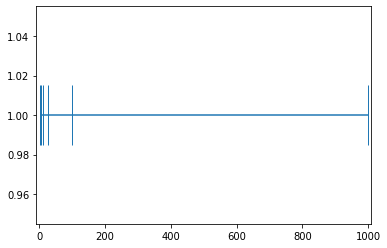How would we transform these numbers to have a mean of 0? Let’s start by finding the current mean of these numbers.

``example_1.mean()``
``190.16666666666666``

190.16666 is the mean of this set of numbers. In order to make this 0, we should be able to just subtract each number by that amount.

``example_1_mean_0 = example_1-example_1.mean(); example_1_mean_0``
``````array([-189.16666667, -185.16666667, -180.16666667, -165.16666667,
-90.16666667,  809.83333333])``````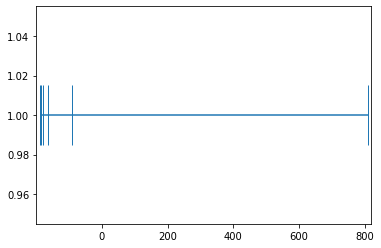``f"The mean is now: {example_1_mean_0.mean()} (AKA: 0)"``
``'The mean is now: 1.8947806286936004e-14 (AKA: 0)'``

Instead of being distributed around the number 190.1666, the numbers are now distributed around the number 0. With the numbers now balanced correctly, we want to remove scale and units from our dataset. statista.com has the following example to explain standard deviation: > 1,000 people were questioned about their monthly phone bill. The mean value is \\$40 and the standard deviation 27. Which means that the average distance of all answers (=values) to the mean is \\$27.

Let’s consider what it means then to divide by the standard deviation. For this example, we would divide by 27 so that the standard deviation is 1 and that is going put us into the same scale whether we started with a huge scale like monthly mortgage amount or monthly phone bill. It strips the original scaling away from the dataset and puts onto a scale where 1 is the distance from the center point. This is helpful because it means that a step in any direction will change the loss the same amount. Another way of putting this is that it smooths the loss landscape so all attributes have the same amount of pull.

``example_1_mean_0_std_1 = example_1_mean_0/example_1_mean_0.std(); example_1_mean_0_std_1``
``````array([-0.52008301, -0.50908566, -0.49533897, -0.45409891, -0.2478986 ,
2.22650516])``````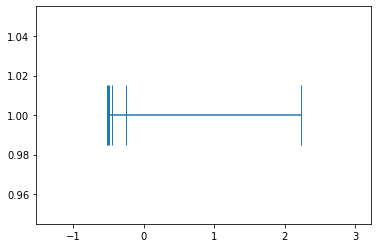``example_1_mean_0_std_1.mean(), example_1_mean_0_std_1.std()``
``(7.401486830834377e-17, 1.0)``

Notice that in all three graphs, the distribution looks the same. Lots of numbers on the left-hand side and only the one number on the right. But to our neural network, there is a huge difference between taking numbers ranging from -0.52 and 2.23 and taking numbers ranging from 1 to 1000. The weights can make a lot finer adjustments on the normalized version and each incoming data point will be on the same scale. That’s why when an image model has been pretrained on Imagenet, you have to keep the same standard deviation and mean. By using those numbers, you are taking all of the pixels in your image and converting them to the normal range for Imagenet.

## Testing the Theory with fastai’s Tabular Application

Now that we have an idea of what is happening, let’s see if we can prove that it actually works how we anticipate. To do this, I will be creating a simple tabular learner and a sample dataloader. I will first train the model on an normalized input and then train the same model using the un-normalized input. My assumption is that the normalized inputs will be much better at converting the x value into y than the un-normalized version.

### Experiment Setup

x1 + xN = y

``````from fastai.tabular.all import *
x = torch.rand((200000,10))
scale_amt = torch.randint(0,100,size=(10,))
#x = x*scale_amt
df = pd.DataFrame(x, columns=[f"x{n}" for n in range(1,11)])
#df['x1']=df['x1']*1000
#df.columns
#df['y'] = df['x1'] + df['x2'] + df['x3'] + df['x4'] + df['x5'] + df['x6'] + df['x7'] + df['x8'] + df['x9'] + df['x10']
df['y'] = (df.values*scale_amt.tolist()).sum(axis=1)
splits = RandomSplitter()(df)
x1 x2 x3 x4 x5 x6 x7 x8 x9 x10 y
0 0.075054 0.456406 0.745085 0.930838 0.109559 0.498177 0.565746 0.743462 0.893542 0.796160 343.622546
1 0.823718 0.925904 0.418757 0.579081 0.645427 0.502683 0.689346 0.439689 0.281477 0.984495 380.632963
2 0.212132 0.928440 0.068775 0.974991 0.274955 0.107026 0.274451 0.439982 0.731169 0.026224 295.966817
3 0.874167 0.900319 0.221668 0.755159 0.525875 0.861770 0.347837 0.993839 0.466206 0.554722 442.779244
4 0.836071 0.795962 0.009992 0.531759 0.619634 0.196202 0.648332 0.899659 0.893503 0.094362 408.136464

`monitor_parameters` is a hook that can be used to monitor values inside of a model. It is a little bit of a hack, but it works really well for getting a better idea what is happening inside the model.

``````def monitor_parameters(m, i, o):
m.weight_track.append(list(m.parameters()).tolist())
m.bias_track.append(list(m.parameters()).tolist())``````

### Normalized

``````to_normalized = TabularPandas(df, cont_names=['x1', 'x2', 'x3', 'x4', 'x5', 'x6', 'x7', 'x8', 'x9', 'x10'], y_names='y', procs=[Normalize], splits=splits)
learn_normalized = tabular_learner(dls_normalized, layers=[], config=tabular_config(use_bn=False, bn_cont=False))
learn_normalized.lr_find(start_lr=1e-3, end_lr=1000000)``````
``````Setting up after_item: Pipeline:
Setting up before_batch: Pipeline:
``SuggestedLRs(valley=3.981071710586548)``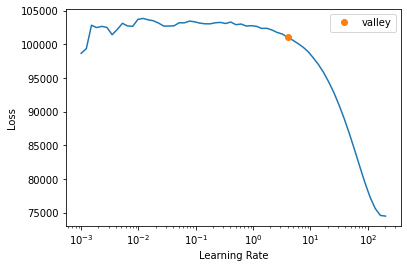``````learn_normalized.model.layers.bias_track = []
learn_normalized.model.layers.weight_track = []
learn_normalized.model.layers.register_full_backward_hook(monitor_parameters)``````
``<torch.utils.hooks.RemovableHandle at 0x7f44a05ccf70>``
``learn_normalized.fit_one_cycle(10, 10)``
epoch train_loss valid_loss time
0 1.142768 0.722641 00:08
1 6.045738 0.614625 00:08
2 16.018417 7.314993 00:08
3 8.353395 5.835221 00:08
4 3.468160 0.493669 00:08
5 2.660133 1.251976 00:08
6 0.722458 0.373421 00:08
7 0.367568 0.272525 00:08
8 0.025171 0.010871 00:08
9 0.000455 0.000518 00:08
``learn_normalized.recorder.plot_loss(skip_start=1000)``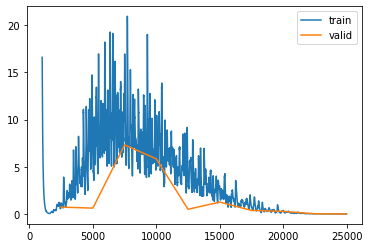``learn_normalized.show_results(ds_idx=0, shuffle=False)``
x1 x2 x3 x4 x5 x6 x7 x8 x9 x10 y y_pred
0 1.562167 -1.569042 -1.114254 0.678972 -0.447562 -0.721848 0.950705 -0.506051 1.427110 1.305289 318.861755 318.871887
1 -0.558561 1.409515 -1.694918 0.051202 -1.187221 0.865891 1.003036 -0.675074 1.489744 -1.504115 319.647339 319.605621
2 0.580085 1.621262 1.555035 -1.474297 0.485046 1.653549 -1.121346 0.184075 1.324292 -0.353307 442.348450 442.299805
3 1.634533 1.501764 0.694681 -0.052227 0.998929 -0.802163 0.824090 1.452214 0.217072 -0.867735 456.612335 456.587646
4 0.305931 0.446660 0.606511 0.404355 -0.881533 -0.470554 1.398422 0.411920 0.697609 1.205599 364.134827 364.130890
5 -0.953506 -1.129230 1.685477 0.200103 1.446620 1.669933 0.654975 -0.218070 0.763460 1.622134 378.366058 378.378632
6 -1.729204 1.402740 1.129965 -1.550283 -0.174763 -1.702276 0.349754 -1.626720 -0.736905 0.554198 219.651688 219.670380
7 1.051801 0.651880 -0.606515 -0.211603 1.065033 1.140438 1.395582 -1.302371 0.548107 0.569542 379.511841 379.522766
8 0.845884 -0.547829 -1.420988 0.976506 -1.266368 -0.449748 -0.053614 -0.578055 0.252759 -0.031057 266.948334 266.938416
``````plt.plot(learn_normalized.model.layers.bias_track)
plt.show()``````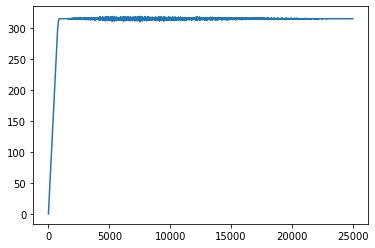``````plt.plot(learn_normalized.model.layers.weight_track)
plt.show()``````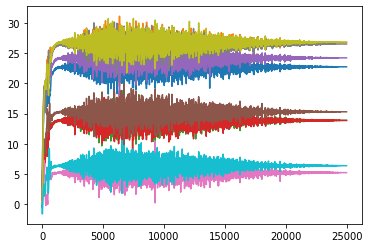### Not Normalized

``````to_not_normalized = TabularPandas(df, cont_names=['x1', 'x2', 'x3', 'x4', 'x5', 'x6', 'x7', 'x8', 'x9', 'x10'], y_names=['y'], splits=splits)
dls_not_normalized.one_batch()
learn_not_normalized = tabular_learner(dls_not_normalized, layers=[], config=tabular_config(use_bn=False, bn_cont=False), train_bn=False)
learn_not_normalized.lr_find(start_lr=1e-3, end_lr=1000000)``````
``````Setting up after_item: Pipeline:
Setting up before_batch: Pipeline:
``SuggestedLRs(valley=3.2359366416931152)``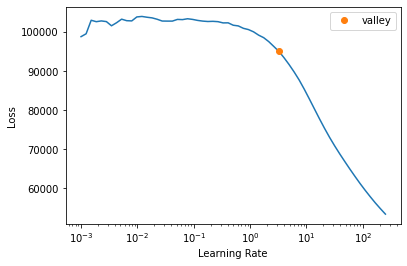``````learn_not_normalized.model.layers.bias_track = []
learn_not_normalized.model.layers.weight_track = []
learn_not_normalized.model.layers.register_full_backward_hook(monitor_parameters)``````
``<torch.utils.hooks.RemovableHandle at 0x7f4473678e80>``
``learn_not_normalized.fit_one_cycle(10, 10)``
epoch train_loss valid_loss time
0 154.066040 153.006943 00:08
1 453.208710 498.198853 00:08
2 450.821014 405.338318 00:08
3 462.034576 507.816345 00:08
4 402.913025 391.320465 00:08
5 299.577545 273.074860 00:08
6 187.224609 182.667740 00:08
7 95.384758 89.960159 00:08
8 57.199078 54.958164 00:08
9 27.854624 28.252810 00:08
``learn_not_normalized.recorder.plot_loss(skip_start=500)``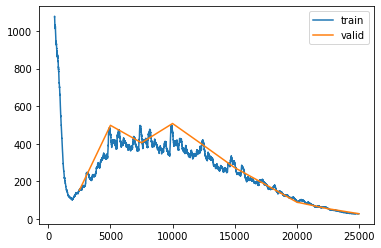``learn_not_normalized.show_results(ds_idx=0, shuffle=False)``
x1 x2 x3 x4 x5 x6 x7 x8 x9 x10 y y_pred
0 0.950224 0.048134 0.178727 0.696215 0.370312 0.293320 0.776244 0.353630 0.912490 0.877288 318.861755 318.913208
1 0.339225 0.906561 0.010991 0.514821 0.156830 0.751503 0.791369 0.304868 0.930580 0.066351 319.647339 318.007935
2 0.667278 0.967587 0.949804 0.074028 0.639484 0.978802 0.177347 0.552729 0.882794 0.398533 442.348450 431.576630
3 0.971073 0.933147 0.701273 0.484935 0.787802 0.270143 0.739647 0.918583 0.563008 0.250043 456.612335 444.280334
4 0.588292 0.629064 0.675804 0.616864 0.245058 0.365837 0.905650 0.618462 0.701796 0.848512 364.134827 360.096039
5 0.225438 0.174889 0.987485 0.557846 0.917016 0.983530 0.690767 0.436712 0.720815 0.968745 378.366058 374.773651
6 0.001953 0.904608 0.827014 0.052072 0.449048 0.010392 0.602547 0.030322 0.287482 0.660485 219.651688 226.905151
7 0.803183 0.688209 0.325397 0.438884 0.806881 0.830731 0.904829 0.123895 0.658617 0.664914 379.511841 374.775940
8 0.743857 0.342450 0.090121 0.782187 0.133986 0.371841 0.485960 0.332858 0.573315 0.491550 266.948334 270.995636
``````plt.plot(learn_not_normalized.model.layers.bias_track)
plt.show()``````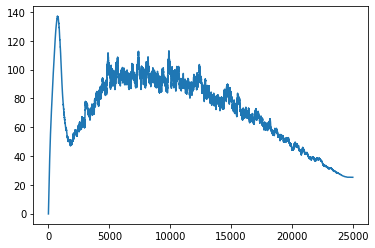``````plt.plot(learn_not_normalized.model.layers.weight_track)
plt.show()``````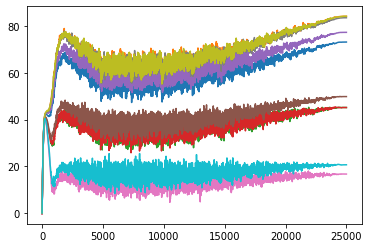``````#actual values the parameter value should have
scale_amt``````
``tensor([79, 93, 48, 48, 84, 53, 18, 92, 93, 22])``
``list(learn_not_normalized.model.layers.parameters())``
``````[Parameter containing:
tensor([[73.1407, 84.1651, 45.1576, 45.1585, 77.2888, 49.8361, 16.7273, 83.5148,
``list(learn_normalized.model.layers.parameters())``
``````[Parameter containing: